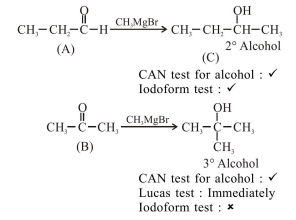# Two compounds A and B with same molecular formula

Question:

Two compounds A and B with same molecular formula $\left(\mathrm{C}_{3} \mathrm{H}_{6} \mathrm{O}\right)$ undergo Grignard's reaction with

methylmagnesium bromide to give products $\mathrm{C}$ and $\mathrm{D}$. products $\mathrm{C}$ and $\mathrm{D}$ show following chemical

tests.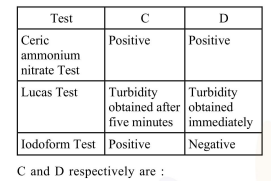1.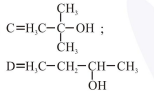2.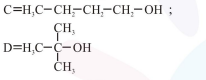3.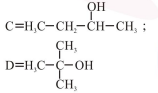4.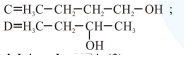Correct Option: , 3

Solution: